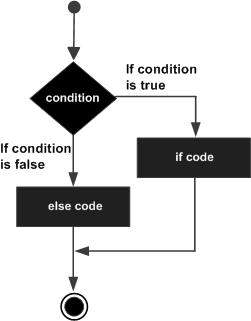# Python 3 - IF...ELIF...ELSE Statements

An else statement can be combined with an if statement. An else statement contains a block of code that executes if the conditional expression in the if statement resolves to 0 or a FALSE value.

The else statement is an optional statement and there could be at the most only one else statement following if.

## Syntax

The syntax of the if...else statement is −

```if expression:
statement(s)

else:
statement(s)
```

## Flow Diagram## Example

```#!/usr/bin/python3

amount = int(input("Enter amount: "))

if amount<1000:
discount = amount*0.05
print ("Discount",discount)
else:
discount = amount*0.10
print ("Discount",discount)

print ("Net payable:",amount-discount)
```

## Output

In the above example, discount is calculated on the input amount. Rate of discount is 5%, if the amount is less than 1000, and 10% if it is above 10000. When the above code is executed, it produces the following result −

```Enter amount: 600
Discount 30.0
Net payable: 570.0

Enter amount: 1200
Discount 120.0
Net payable: 1080.0
```

## The elif Statement

The elif statement allows you to check multiple expressions for TRUE and execute a block of code as soon as one of the conditions evaluates to TRUE.

Similar to the else, the elif statement is optional. However, unlike else, for which there can be at the most one statement, there can be an arbitrary number of elif statements following an if.

### syntax

```if expression1:
statement(s)
elif expression2:
statement(s)
elif expression3:
statement(s)
else:
statement(s)
```

Core Python does not provide switch or case statements as in other languages, but we can use if..elif...statements to simulate switch case as follows −

### Example

```#!/usr/bin/python3

amount = int(input("Enter amount: "))

if amount<1000:
discount = amount*0.05
print ("Discount",discount)
elif amount<5000:
discount = amount*0.10
print ("Discount",discount)
else:
discount = amount*0.15
print ("Discount",discount)

print ("Net payable:",amount-discount)
```

When the above code is executed, it produces the following result −

```Enter amount: 600
Discount 30.0
Net payable: 570.0

Enter amount: 3000
Discount 300.0
Net payable: 2700.0

Enter amount: 6000
Discount 900.0
Net payable: 5100.0
```
python_decision_making.htm